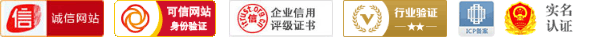## 大盘相关系数指标及源代码Beta系数是用以度量一项资产系统性风险(systematic risk)的指标，是资本资产定价模型(Capital Asset Pricing Model)的主要参数。它的作用是衡量一种证券或一个投资组合(asset allocation)相对总体市场的波动性的一种证券系统性风险的评估工具。

β > 1, 即证券价格比总体市场更波动(效市场为高风险公司或投资)。
β < 1, 即证券价格的波动性比市场为低(效市场为低风险公司或投资)。
β = 0, 即证券价格的波动与市场没有关系。
β < 0, 即证券价格的波动与市场为相反, 一般情况下很少见。

Beta是通过统计分析同一时期市场每天的收益情况以及单个股票每天的价格收益来计算出的。当Beta值处于较高位置时，投资者便会因为股份的风险高而会相应提升股票的预期回报率。

n:=250;
x:=(CLOSE-CLOSE)/CLOSE*100;
r:=INDEXC;
y:=(r-r)/r*100;
BETA:(n*SUM(x*y,n)-SUM(x,n)*SUM(y,n))/(n*SUM(y*y,n)-POW(SUM(y,n),2));

------分隔线----------------------------@2017 CFTSC 操盘手联盟工作室，欢迎您的加入，携手共创美好明天。